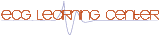The 12 Lead ECG and Method of Interpretation (Lessons I and II)

## Question 1: Determine the QRS axis for this ECG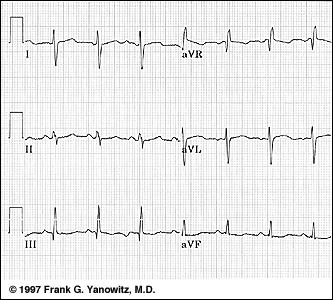A. Indeterminate
B. +90 degrees
C. -30 degrees
D. -45 degrees
E. +150 degrees

## Question 2: Determine the axis for this ECG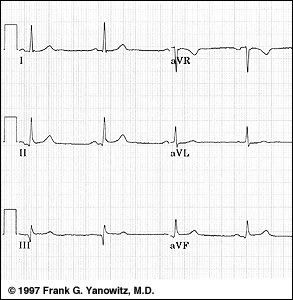A. +90 degrees
B. Indeterminate
C. +30 degrees
D. -30 degrees
E. -150 degrees

## Question 3: What is the normal range of the QRS axis?

A. 0 degrees to 180 degrees
B. 0 degrees to +90 degrees
C. -30 degrees to +90 degrees
D. -90 degrees to +90 degrees
E. -90 degrees to +30 degrees

## Question 4: What direction of current do leads V1 and V2 measure?

A. Left / Right forces
B. Leftward forces only
C. Anterior / Posterior forces
D. Superior / Inferior forces
E. Inward / Outward forces

A. aVF
B. II
C. III
D. V1
E. V6

## Question 6: Determine the QRS axis for this ECG: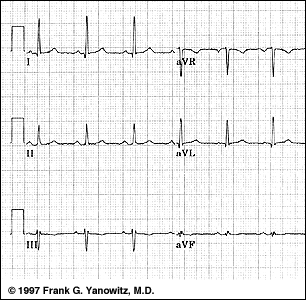A. -60 degrees
B. -45 degrees
C. +60 degrees
D. Indeterminate
E. 0 degrees

## Question 7: Determine the QRS axis for this ECG: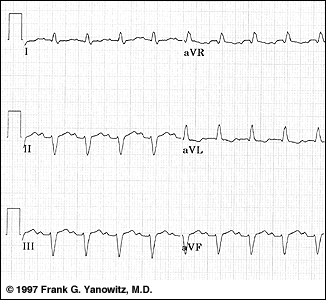A. -75 degrees
B. -30 degrees
C. 0 degrees
D. +45 degrees
E. Indeterminate

## Question 8: Determine the QRS axis for this ECG: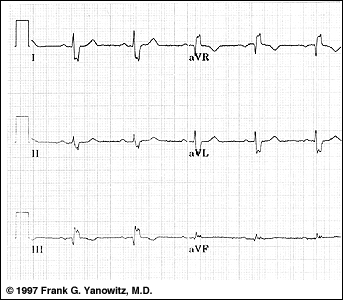A. -15 degrees
B. +15 degrees
C. +60 degrees
D. +105 degrees
E. Indeterminate

## Question 9: Determine the QRS axis for this ECG: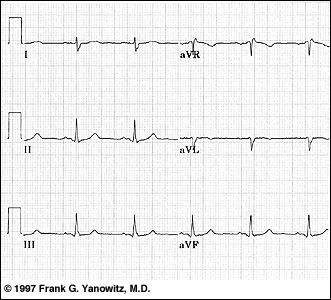A. -100 degrees
B. -30 degrees
C. +15 degrees
D. +90 degrees
E. Indeterminate

A. Lead I
B. aVF
C. aVL
D. V1
E. V6